# How to calculate Sum of Digits in a Number using Non Array Formulas in Excel?

• Last Updated : 01 Jul, 2022

In Excel, we can easily add the digits of a number. Usually, calculations that involve multiple values can be dealt with the help of array formulas, but here, we are going to find the sum of the digits of a number using non-array formulas. As an example, say we have a number 238 in one of the cells of the Excel sheet and we want to find the sum of the digits of this number. It would essentially be 2 + 3 + 8 which equals 13. Let us see how to do that without using an array formula. But before jumping on to the non-array formula method of finding the sum of the digits of a number, let us first see what is an array formula.

### Array Formula

An array formula is nothing but a method that enables one to do multiple calculations at once. It can also do a calculation more than once in a specific cell range. Look at the example below. Say we have to find the product of quantities and cost as shown in the excel sheet below. If we do this using an array formula, then we can get the result much faster.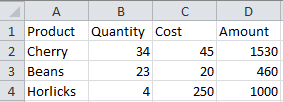We can use the multi-cell array formula for multiplying the quantity and cost column.

Multi-cell array formula

Here, we have to use a single formula and we will get results of all the rows. To calculate all the values of the Amount table, we will first select the area where we want to see the end result. Press F2 after selecting the required area so that you come back on to the first cell of the selected area.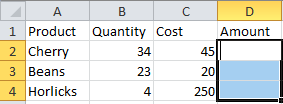Then, write the array formula in the first cell where the cursor is placed. The formula is simply: =B2:B4*C2:C4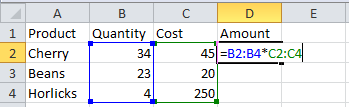Now, don’t just press enter because then it will print the result in only one cell. These formulas are CSE, meaning they will execute properly only when you click CTRL + SHIFT + ENTER. See the result.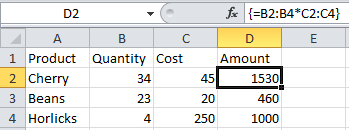Note that Excel automatically adds curly braces around the formula. This is to indicate that it is an array formula.

### Non-array Formula

There are two non-array formulas that we can use to find the sum of the digits of a number. These are:

1. Sum and Index Formula
2. Sumproduct and Indirect Formula

Let us look at them one by one.

1. Sum and Index formula: Here, we have a number 243 and we have to find the sum of its digits. We will go to the cell in which we want to get the sum and write the following formula:

=SUM(INDEX(1*(MID(A1,ROW(INDIRECT(“1:”&LEN(A1))),1)),,))

This formula uses various functions. Let us understand this formula a bit more:

• The INDEX function will return the value at a given location. In this case, it would be the value of these numbers.
• The MID function will return a sequence of values from the middle of the string. In this case, the string is nothing but the number itself.
• The ROW function will simply return the number for the referenced. Here the row number will be A1.
• The INDIRECT function will return a valid cell reference from this string of numbers.
• The LEN function will return the number of characters of the string. In this case, it would be 3.

Sum and Index formula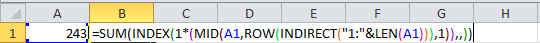Just press Enter and you will get the result.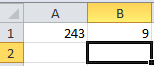2. Sumproduct and Indirect Formula: Again we have a number 243 and we have to find the sum of its digits. We will go to the cell in which we want to get the sum and write the following formula:

=SUMPRODUCT(MID(A1,ROW(INDIRECT(“1:” & LEN(A1))),1)*1)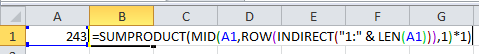There is one more formula under this category that works the same:

=SUMPRODUCT(MID(A1,ROW(OFFSET(\$A\$1,,,LEN(A1))),1)+0)

It is shown here: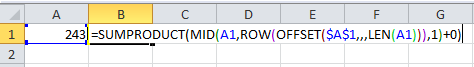Both these formulae give the same output:A few more functions are used in this formula. These are:

• The OFFSET function will return a range specifying a certain number of rows and columns to address a cell.
• The SUMPRODUCT function first multiplies a range of cells and then returns the sum of the product.
My Personal Notes arrow_drop_up
Recommended Articles
Page :